﻿ Some-Dogtone-Fuzztones

Some Fuzztones Made By Dogtone

Some With Sound Samples (click a pic)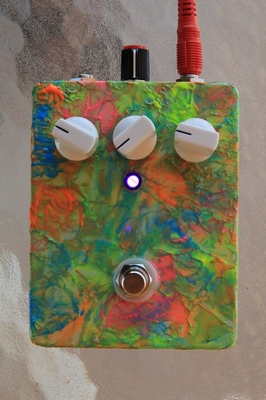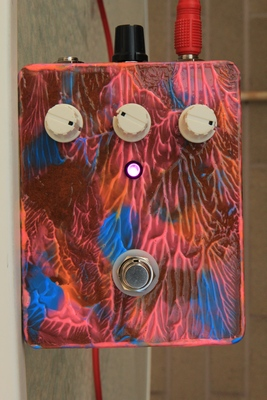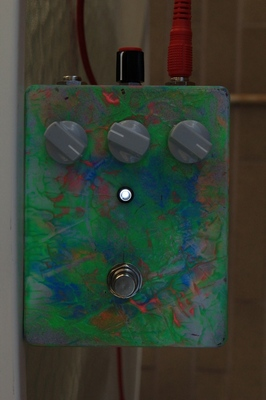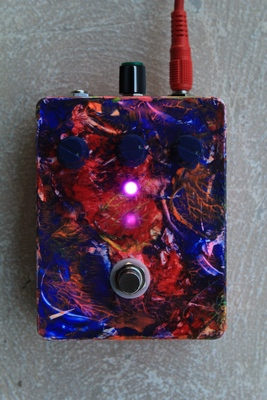Q1 = GT308B : Q2 = GT308B Q1 = p416b : Q2 = p416b Q1 = GT308B : Q2 = GT308B Q1 = p416b : Q2 = p416b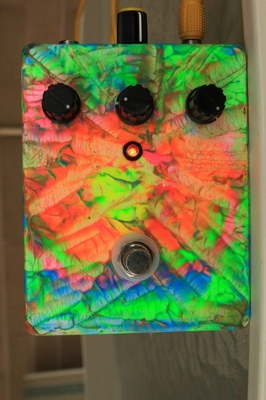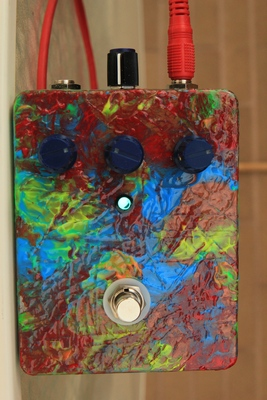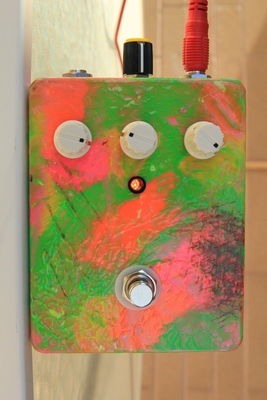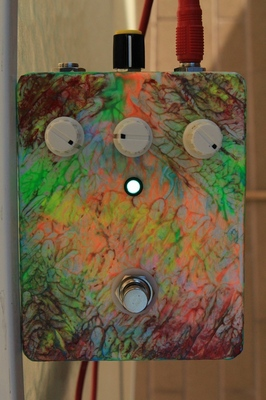Q1 = GT308B : Q2 = GT402b Q1 = GT402J : Q2 = p416b Q1 = GT308B : Q2 = p416b Q1 = p416b : Q2 = GT308B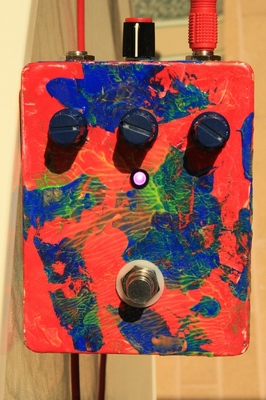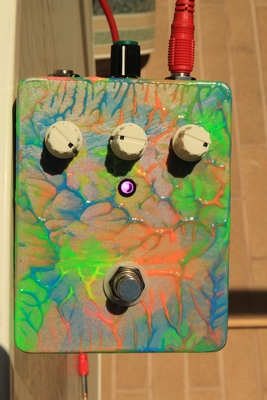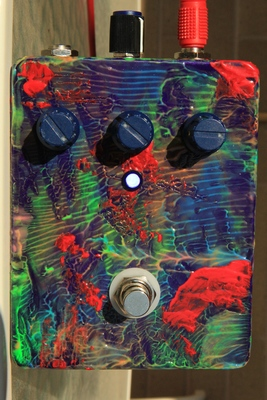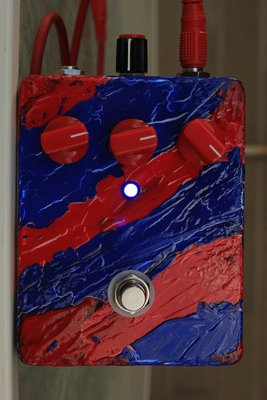Q1 = p416b : Q2 = p416b Q1 = p416b : Q2 = GT308B Q1 = p416b : Q2 = GT308B Q1 = GT308B : Q2 = p416b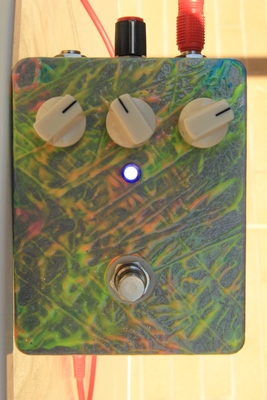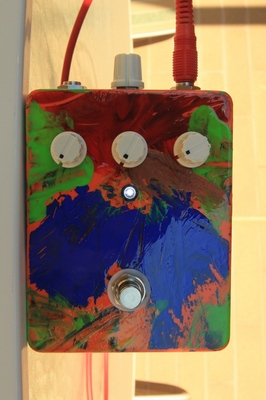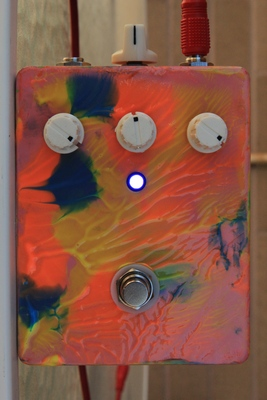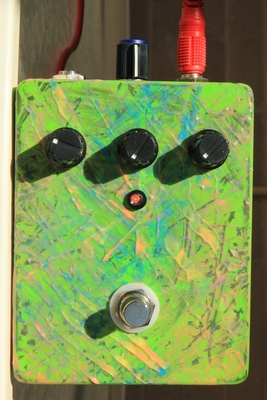Q1 = GT308B : Q2 = p416b Q1 = p416b : Q2 = GT308B Q1 = p416b : Q2 = GT308B Q1 = GT308B : Q2 = p416b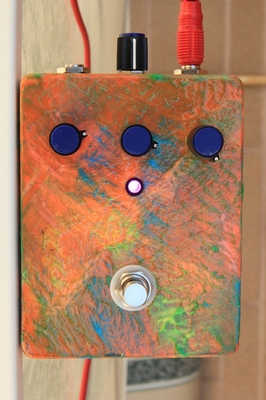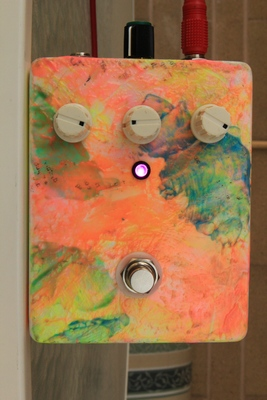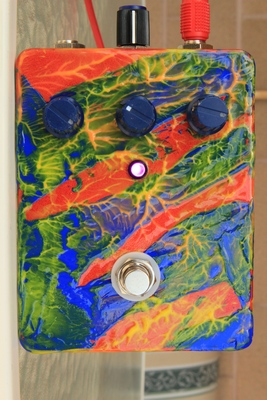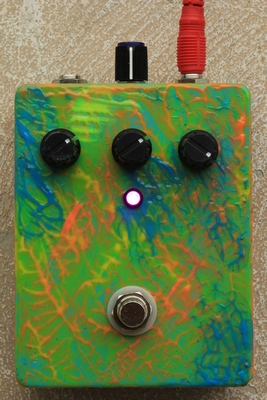Q1 = GT308B : Q2 = p416b Q1 = GT402J : Q2 = GT308B Q1 = GT308B : Q2 = p416b Q1 = GT308B : Q2 = p416b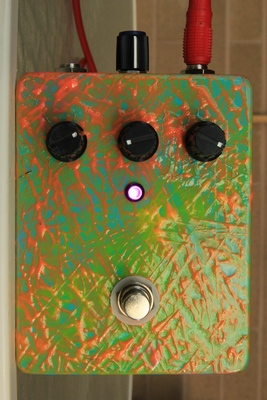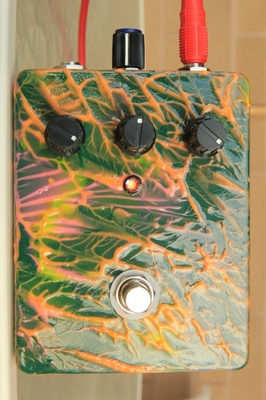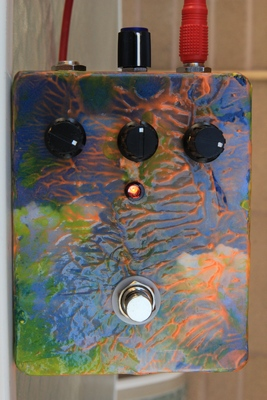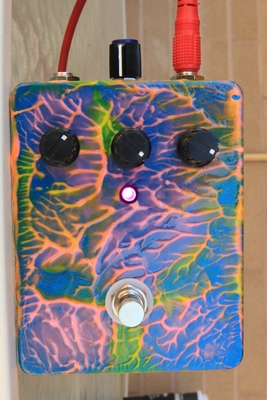Q1 = GT308B : Q2 = p416b Q1 = GT308B : Q2 = p416b Q1 = GT308B : Q2 = p416b Q1 = p416b : Q2 = GT308B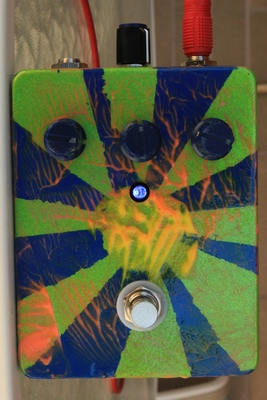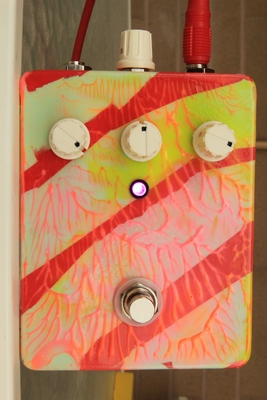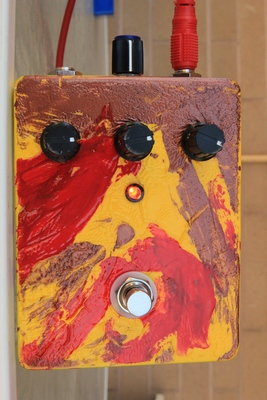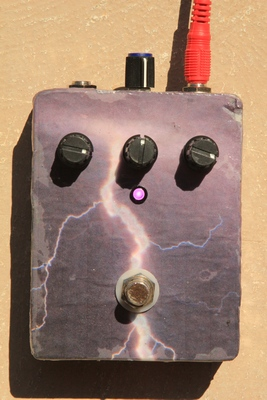Q1 = GT308B : Q2 = p416b Q1 = GT308B : Q2 = p416b Q1 = GT308B : Q2 = p416b Q1 = GT308B : Q2 = p416b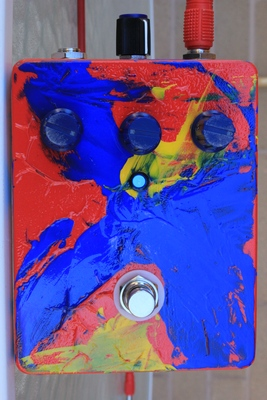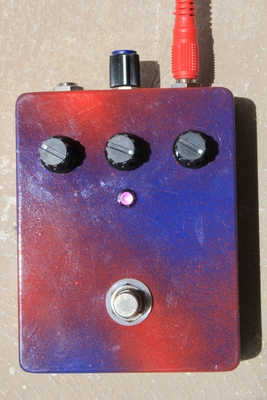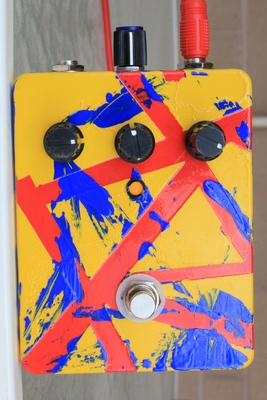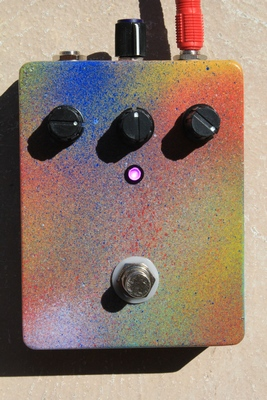Q1 = p416b : Q2 = GT308B Q1 = GT308B : Q2 = p416b Q1 = TI CV7003 : Q2 = NTK271 Q1 = GT308B : Q2 = p416b